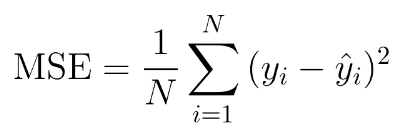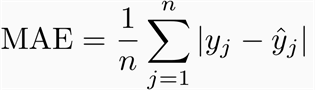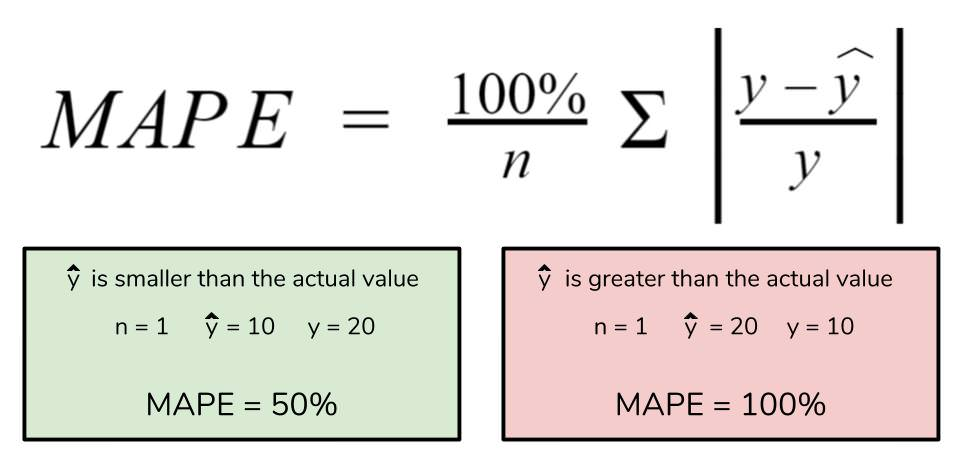Model Metric Definitions
Definitions for common model metrics

## Accuracy

Accuracy is the measure of the number of correct predictions made by the model. It is derived by calculating the percentage of correct predictions out of overall predictions.
Accuracy = correct predictions / all predictions
Example: There are 100 credit card transactions; 90 transactions are legitimate and 10 transactions are fraudulent. If your model predicts that 95 transactions are legitimate and 5 transactions are fraudulent, its accuracy is:
95% = (90 correct legitimate + 5 correct fraudulent) / 100 transactions

## Precision

Precision is the fraction of values that actually belong to a positive class out of all the values which were predicted to belong to that class
precision = true positives / (predicted true positives + predicted false positives)
Example: There are 100 credit card transactions; 80 transactions are legitimate (positive class) and 20 transactions are fraudulent. If your model predicts that 85 transactions are legitimate, its precision is:
94.12% = 80 true positives / (80 true positives + 5 false positives)

## Recall

Recall is the fraction of values predicted to be of a positive class out of all the values that truly belong to the positive class (including false negatives)
recall = predicted true positives / (true positives + false negatives)
Example: There are 100 credit card transactions; 90 transactions are legitimate (positive class) and 10 transactions are fraudulent. If your model predicts that 80 transactions are legitimate and 20 transactions are fraudulent, its recall is:
88.89% = 80 true positives / (80 true positives + 10 false negatives)

## KL Divergence

The Kullback-Leibler Divergence metric is calculated as the difference between one probability distribution from a reference probability distribution. KL divergence is sometimes referred to as ‘relative entropy’ and best used when one distribution is much smaller in sample and has a large variance.

## Population Stability Index (PSI)

Population Stability Index looks at the magnitude which a variable has changed or shifted in distribution between two samples over the course of a given time. PSI is calculated as:
PSI = (% Actual - % Expected) x ln(% Actual / % Expected)
The larger the PSI, the less similar your distributions are, which allows you to set up thresholding alerts on the drift in your distributions. PSI is a great metric for both numeric and categorical features where distributions are fairly stable.

## RMSE

Root Mean Square Error (also known as root mean square deviation, RMSD) is a measure of the average magnitude of error in quantitative data predictions. It can be thought of as the normalized distance between the vector of predicted values and the vector of observed (or actual) values.Because errors are squared before averaged, this measure gives higher weight to large errors, and therefore useful in cases where you want to penalize models accordingly.

## MSE

Mean Square Error a regressive loss measure. The MSE is measured as the difference between the model’s predictions and ground truth, squared and averaged out across the dataset. It is used to check how close the predicted values are to the actual values. As in RMSE, a lower value indicates a better fit, and it heavily penalizes large errors or outliers.## MAE

Mean Absolute Error is a regressive loss measure looking at the absolute value difference between a model’s predictions and ground truth, averaged out across the dataset. Unlike MSE, MAE is weighted on a linear scale and therefore doesn’t put as much weight on outliers. This provides a more even measure of performance, but means large errors and smaller errors are weighted the same. Something to consider depending on your specific model use case.## MAPE

Mean Absolute Percentage Error is one of the most common metrics of model prediction accuracy and the percentage equivalent of MAE. MAPE measures the average magnitude of error produced by a model, or how far off predictions are on average. See MAE for considerations when using this metric.## NDCG

NDCG measures a model's ability to rank query results in the order of the highest relevance. Actual relevance scores are usually determined by user interaction. For example, if users tend to click on results ranked high on the list, then the NDCG value will be high. Conversely, if users tend to click on query results that are ranked low on the list, it would mean that the ranking model is doing poorly, and the NDCG value will be low. NDCG values range between 0 and 1 with 1 being the highest. Arize computes NDCG using the standard log2 discount function.

## F-score

Measure of the harmonic mean of precision and recall. F-score is a result of integrating these parameters into one for a better understanding of the accuracy of the model. F-score can be modified into F, 0.5, 1, & 2 based on the measure of weightage given to precision over recall.

## Logarithmic Loss

Tracks incorrect labeling of the data class by the model and penalises the model if deviations in probability occur into classifying the labels. Low log loss values equate to high accuracy values.

## Performance Impact Score

Performance impact score is a measure of how much worse your metric of interest is on the slice compared to the average.

## PR Curve

The Precision-Recall curve is the correlation between the precision and recall at particular cut-off values, with the cut off values being set according to the particular model.

## ROC - AUC

The Receiver Operating Characteristics (ROC) is a probability curve plotted between true positive rate (TPR) and false positive rate (FPR). Area Under the Curve (AUC) is an aggregate measure of performance across all possible classification thresholds. Together, ROC - AUC represents the degree of separability, or how much a model is capable of distinguishing between classes.
The higher the AUC (i.e. closer to 1), the better the model is at predicting 0 class as 0, and 1 class as 1.
FPR = X = fp / ( tn + fp) => error: when all actuals are negative
TPR = Y = tp / (tp + fn) => error: when all actuals are positiveTo calculate AUC/PR AUC: Threshold: We first need to generate a confusion matrix based on the threshold. There are 20 thresholds by default , so there will be 20 confusion matrices as well.
From each confusion matrix: we calculate the False Positives Rate (x-axis) and True Positive Rate (y-axis) for AUC. As a result, we will have a set of 20 points (x and y coordinates) for AUC or PR-AUC.
AUC: The next step is to calculate the area under the curve (AUC) for the 20 points. We first sort the points in order of x-axis increasing, and then a secondary sort on y-axis to make it consistent between runs. According to trapezoidal rule, we find the difference between x values of two consecutive points as delta x, and multiply by the average of y values of two consecutive points to get the area between two consecutive points. Lastly, we do the cumulative sum for all 20 points to get the overall AUC.

## Sensitivity

Sensitivity is a measure of the number of positive cases that turned out to be true for a given model. It is also called the true positive rate.
sensitivity = predicted true positives / (true positives + false negatives)

## Specificity

Specificity is the fraction of values predicted to be of a negative class out of all the values that truly belong to the negative class (including false positives). This measure is similar to recall, except it describes the offset in correcting predicted negative values. It is also called the true negative rate.
specificity = predicted true negatives / (true negatives + false positives)
Example: There are 100 credit card transactions; 90 transactions are legitimate and 10 transactions are fraudulent (negative class). If your model predicts that 20 transactions are fraudulent, its recall is:
50% = 10 true negatives / (10 true negatives + 10 false positives)

## Calibration

Calibration is the comparison of the actual output and the expected output given by a system.
Example: Let's say we are predicting credit card fraud and produce the following prediction/actual pairs `(0.1, 0.1), (0.2, 0.1), (0.9, 0.7)`.
Our calibration calculation is as follows:
calibration = average prediction / average actual
= `((0.1 + 0.2 + 0.9)/3) / ((0.1 + 0.1 + 0.7)/3) = 0.4 / 0.3 `
` = 1.333`
In this case we see we are on average predicting higher (more fraud) than the ground truth.

## WAPE

Weighted Average Percentage Error, also also referred to as the MAD/Mean ratio. The WAPE metric is the sum of the absolute error normalized by the sum of actual values. WAPE equally penalizes for under-forecasting or over-forecasting, and does not favor either scenario.
`WAPE = sum(absError)/sum(actuals)`
When the total number of sales can be low or the product analyzed has intermittent sales, WAPE is recommended over MAPE. MAPE is commonly used to measure forecasting errors, but it can be deceiving when sales reach numbers close to zero, or in intermittent sales (referenced here). WAPE is a measure that counters this by weighting the error over total sales. WAPE is more robust to outliers than Root Mean Square Error (RMSE) because it uses the absolute error instead of the squared error.